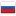Codeforces Round #316 Editorial

Revision en1, by josdas, 2015-08-13 23:37:55

570А — Elections

We need to determine choice for each city. Then sum it for each candidate and determine the winner.

O(n * m)

570B — Simple Game

Lets find which variant is interesting. For Andrew is no need a variant wherein |a-m|>1 because we can increase probability of victory if we will be closer to m. Then we consider two variants,a=c-1 and a=c+1. Probability of victory will be (c-1)/n for first variant and (n-c+1)/n for second.

We need to choose better variant, also we must keep in mind case of n=1.

O(1)

570C — Replacement

Lets find how replacements occur. If we have segment of points with length l,we need l-1 operations and stop replacements for this segment. If we sum lenghts of all segments and its quantity then answer will be = total length of segments — quantity of segments. After change of one symbol length changes by 1.

Quantity of segments can be supported by array. Consider events of merging, dividing,creation and deletion of segments. For merging we need to find if both of neighbors(right and left) are points then merging occured and quantity of segments reduced by 1. Other cases can be cosidered similarly.

O(n + m)

570D — Tree Requests

We need to write vertices in DFS order and store time of enter/exit of vertices in DFS. All vertices in subtree represent a segment. Now we can get all vertices in subtree v on height h as a segment, making two binary searches.

We can make a palindrome if quantity of uneven entries of each letter is less than 2.

This function can be counted for each prefix in bypass for each depth.

For saving the memory bit compression can be used considering that we need only parity and function is xor.

O(m * (log n + 26) + n)

570E — Pig and Palindromes

We need palindrome paths. Palindrome is word which reads the same backward or forward. We can use it. Count the dynamic from coordinates of 2 cells, first and latest in palindrome.

From each state exists 4 transitions (combinations: first cell down/to the right and second cell up/to the left). We need only transitions on equal symbols for making a palindrome. Note that we need a pairs of cells on equal distance from start and end for each.

For saving memory we need to store two latest layers.

O(n^3)-time and O(n^2)-memory

#### History

RevisionsRev. Lang. By When Δ Comment
en4josdas 2015-08-15 08:10:02 4 Tiny change: ' will be $(c-1)/n$ for fi' -> ' will be $c/n$ for fi'
ru4josdas 2015-08-15 08:08:58 6 Мелкая правка: 'м случае $(c – 1) / n$, во ' -
en3josdas 2015-08-14 09:39:01 91
en2josdas 2015-08-14 09:35:48 650 Tiny change: '(m * (log n + 26) + n' -
ru3josdas 2015-08-14 09:17:53 741 Мелкая правка: '(Div. 2)
en1josdas 2015-08-13 23:37:55 2851 Initial revision for English translation
ru2josdas 2015-08-13 22:14:27 14
ru1josdas 2015-08-13 22:12:07 3252 Первая редакция (опубликовано)